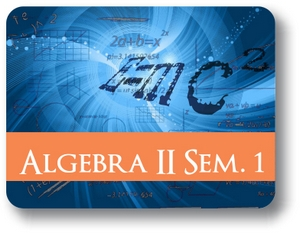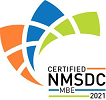Red Comet## Algebra II - Semester - 1

#### Course Price: \$250.00## Course Details:

This course helps the student to understand the meaning, significance and objectives of Algebra. The different types of functions, including exponential and logarithmic are described. The student acquires the knowledge and skills necessary to understand the principles and requirements of solving various problems using permutations and combinations. Student explores the relevance and applications of understanding word problems and interpreting them in terms of complex equations. Student understands how to interpret inverse variations and write the correct equations. This course demonstrates to the student how the same algebraic techniques can be applied to various situations. Students build upon what they had learned in Algebra I and solve more complex problems.

## Syllabus:

Section 1: Functions

• Recap: Functions
• Combining Functions
• Composite Functions
• Transforming Functions
• Inverse Functions

Section 2: Complex Numbers

• Imaginary numbers
• Complex Numbers
• Complex Plane
• Adding and Subtracting Complex numbers
• Multiplying and Dividing Complex Numbers

Section 3: Polynomials

• Recap: Arithmetic with Polynomials
• The Binomial Theorem
• Factoring Polynomials
• The Fundamental Theorem of Algebra
• Finding Zeros of Polynomials
• Graphs of Polynomials

• Solving Equations Containing Square Root
• Extraneous Solutions
• Solving Radical Equations with nth Roots
• Rational Exponents

Section 5: Rational Expressions

• Simplifying Rational Expressions
• Arithmetic with Rational Expressions
• Solving Rational Equations
• Graphs of Rational Equation

### Accreditation & Approvals